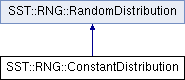SST  11.1.0 StructuralSimulationToolkit
SST::RNG::ConstantDistribution Class Reference

Implements a distribution which always returns a constant value (provided by the user). More...

`#include "sst/core/rng/constant.h"`

Inheritance diagram for SST::RNG::ConstantDistribution:## Public Member Functions

ConstantDistribution (double v)
Creates a constant distribution which returns a constant value. More...

~ConstantDistribution ()
Destroys the constant distribution.

double getNextDouble ()
Gets the next double for the distribution, in this case it will return the constant value specified by the user. More...

double getMean ()
Gets the constant value for the distribution. More...Public Member Functions inherited from SST::RNG::RandomDistribution
virtual ~RandomDistribution ()
Destroys the distribution.

RandomDistribution ()
Creates the base (abstract) class of a distribution.

## Protected Attributes

double mean
Describes the constant value to return from the distribution.

## Detailed Description

Implements a distribution which always returns a constant value (provided by the user).

This can be used in situations where the user may not want to apply a distribution.

## Constructor & Destructor Documentation

 SST::RNG::ConstantDistribution::ConstantDistribution ( double v )
inline

Creates a constant distribution which returns a constant value.

Parameters
 v Is the constant value the user wants returned by the distribution

## Member Function Documentation

 double SST::RNG::ConstantDistribution::getMean ( )
inline

Gets the constant value for the distribution.

Returns
Constant value specified by the user when creating the class
 double SST::RNG::ConstantDistribution::getNextDouble ( )
inlinevirtual

Gets the next double for the distribution, in this case it will return the constant value specified by the user.

Returns
Constant value specified by the user when creating the class

Implements SST::RNG::RandomDistribution.

The documentation for this class was generated from the following file: